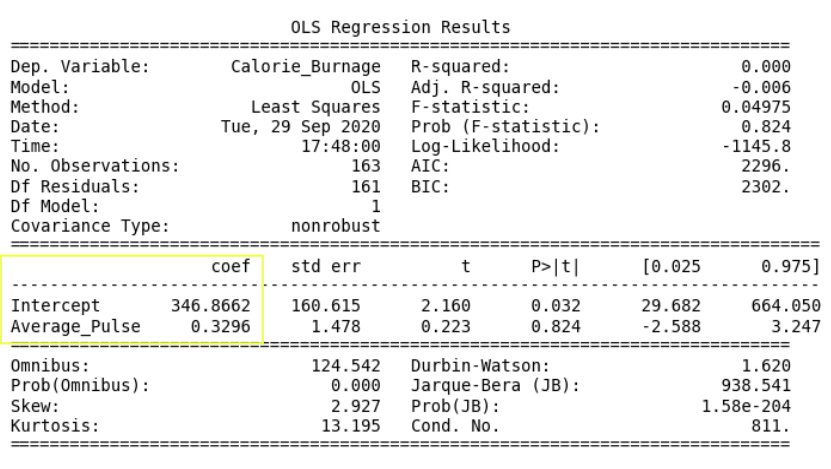# Data Science - Regression Table - Coefficients

## The "Coefficients Part" in Regression Table• Coef is short for coefficient. It is the output of the linear regression function.

The linear regression function can be rewritten mathematically as:

Calorie_Burnage = 0.3296 * Average_Pulse + 346.8662

These numbers means:

• If Average_Pulse increases by 1, Calorie_Burnage increases by 0.3296 (or 0,3 rounded)
• If Average_Pulse = 0, the Calorie_Burnage is equal to 346.8662 (or 346.9 rounded).
• Remember that the intercept is used to adjust the model's precision of predicting!

Do you think that this is a good model?

## Define the Linear Regression Function in Python

Define the linear regression function in Python to perform predictions.

What is Calorie_Burnage if Average_Pulse is: 120, 130, 150, 180?

### Example

def Predict_Calorie_Burnage(Average_Pulse):
return(0.3296*Average_Pulse + 346.8662)

print(Predict_Calorie_Burnage(120))
print(Predict_Calorie_Burnage(130))
print(Predict_Calorie_Burnage(150))
print(Predict_Calorie_Burnage(180))
Try it Yourself »

W3Schools is optimized for learning and training. Examples might be simplified to improve reading and learning. Tutorials, references, and examples are constantly reviewed to avoid errors, but we cannot warrant full correctness of all content. While using W3Schools, you agree to have read and accepted our terms of use, cookie and privacy policy.